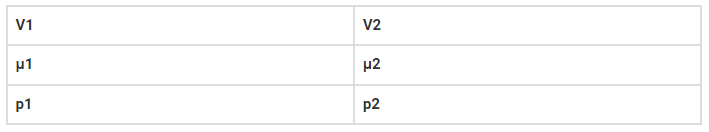# The container shown in the figure has two chambers,`
Question:

The container shown in the figure has two chambers, separated by a partition, of volumes V1 = 2.0 litre and V2 = 3.0 litre. The chambers contain μ1 = 4.0 and μ2 = 5.0 moles of a gas at pressure p1 = 1.00 atm and p2 = 2.00 atm. Calculate the pressure after the partition is removed and the mixture attains equilibrium.Solution:

For an ideal gas, PV = μRT

P1V1 = μ1R1T1

P2V2 = μ2R2T2

P1 = 1 atm

P2 = 2 atm

V1 = 2L

V2 = 3L

T1 = T = T2

μ1 = 4

μ2 = 5

Substituting all the values we get, P = 1.6 atm